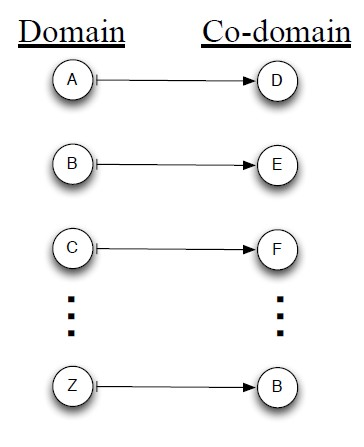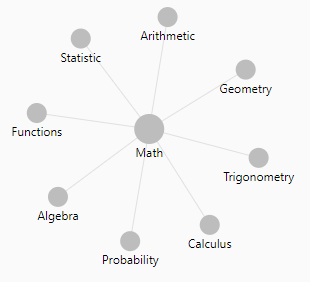# Mathematics - Domain of a function (set of input )

In mathematics, the domain of definition or simply the domain of a function is the set of “input” or argument values for which the function is defined. That is, the function provides an “output” or value for each member of the domain.

In the notation: $$f(A) = B \\ f : A \rightarrow B$$

• X is the domain
• and Y is the codomain.

## Documentation / Reference

Recommended PagesFunction - Scalar

Scalar functions return a single value (as opposed to an array). Mathematics definition: Any function whose domain is a vector space and whose value is its scalar field. Is the result of a scalar function...Linear Algebra - Function (Set)

For each input element in a set , a function assigns a single output element from another set . is called the domain of the function is called the co-domain or in Mathese: The function...Mathematics - codomain of a function (set of output)

In mathematics, the codomain or target set of a function is the set Y into which all of the output of the function is constrained to fall. It is the set Y in the notation f: X → Y. X is the domain....